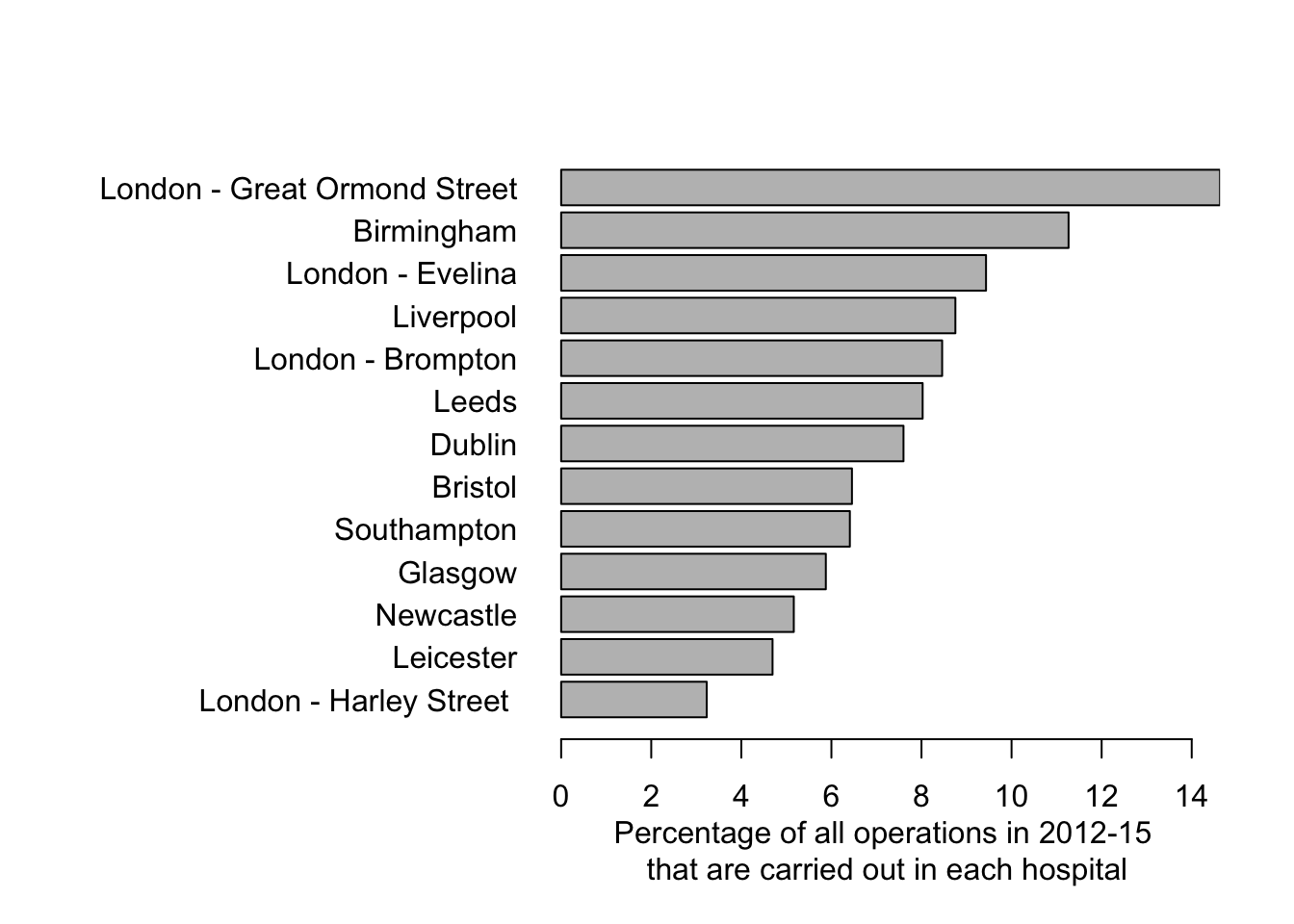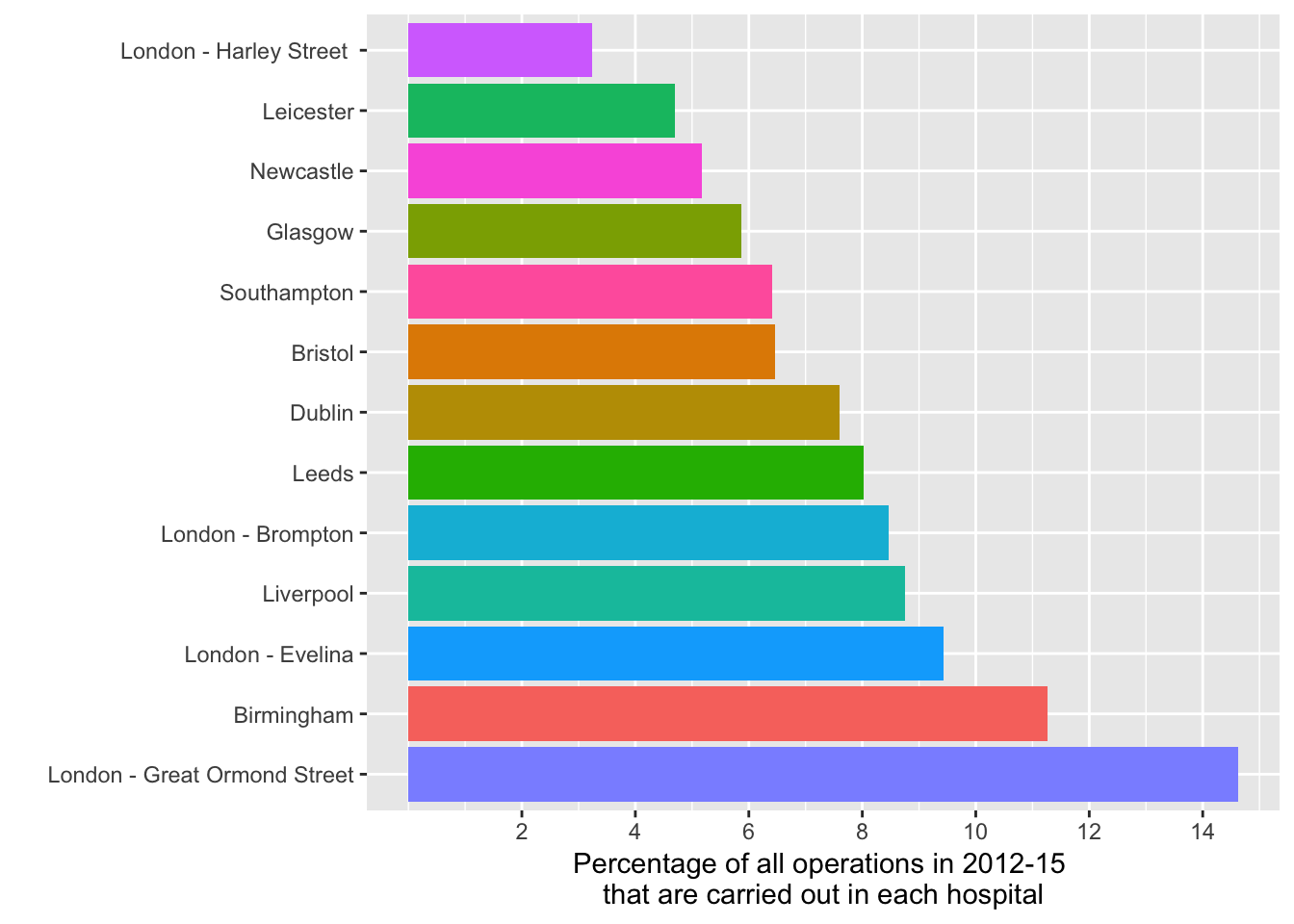Figure 1.3: Percentage of all child heart surgery being carried out in each of thirteen hospitals

Data are shown in Table 1.1 (page 23) and are contained in 01-1-child-heart-survival-x.csv. The data were originally presented in the NCHDA 2012-15 report, but are best seen on childrensheartsurgery.info.

library(ggplot2)

df$Percentage = 100*df$Operations/sum(df$Operations) df$Pos= rank(df$Percentage) First in R base graphics par(mar=c(5,15,4,2)) barplot(df$Percentage,names.arg=df\$Hospital,horiz=T, xpd=F,las=1, xlab="Percentage of all operations in 2012-15 \nthat are carried out in each hospital")Now in ggplot2

bp <- ggplot(df, aes(x=reorder(Hospital,-Pos), y=Percentage, fill=Hospital)) #sets initial plot object from the dataframe for Hospitals, reordered by Percentage (descending) as the y-values, colour-filled by Hospital
bp <- bp + geom_bar(stat = "identity") + labs(x="Hospital") # makes the plot a bar-chart
bp <- bp + coord_flip() # makes it an horizontal bar chart
bp <- bp + scale_y_continuous(breaks=seq(2,16,2)) # breaks every two-count
bp <- bp + theme(legend.position="none") # removes the legend
bp <- bp +  labs(y="Percentage of all operations in 2012-15 \nthat are carried out in each hospital", x="") # Adds labels
bp # draws the plotFigure 1.3 Percentage of all operations in 2012-15 that are carried out in each hospital: a clearer representation using a horizontal bar chart.